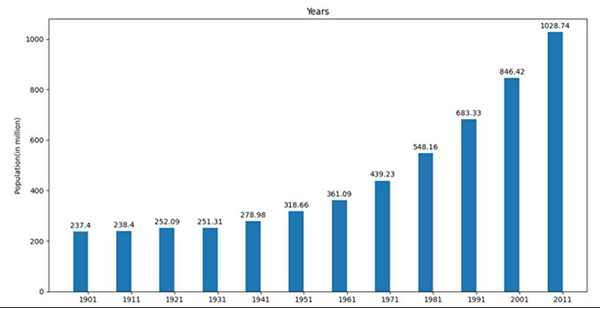# Adding value labels on a matplotlib bar chart

In this program, we can initialize some input values and then try to plot a bar using those values. We can instantiate a figure and axis so that we could set the label, ticks, and annotate the height and width of the bar.

## Steps

• Make a list of years.

• Make a list of populations in that year.

• Get the number of labels using np.arrange(len(years)) method.

• Set the width of the bars.

• Create fig and ax variables using subplots() method, where default nrows and ncols are 1.

• Set the Y-axis label of the figure using set_ylabel().

• Set the title of the figure, using set_title().

• Set the X-ticks with x that is created in step 3, using set_xticks method.

• Set the xtick_labels with years data, using set_xticklabels method.

• Make a plot bar using ax.bar().

• Iterate the bar container (from step 10) to annotate to set the value for every bars.

• Use plt.show() to show the figure.

## Example

from matplotlib import pyplot as plt
import numpy as np

years = [1901, 1911, 1921, 1931, 1941, 1951, 1961, 1971, 1981, 1991, 2001, 2011]
population = [237.4, 238.4, 252.09, 251.31, 278.98, 318.66, 361.09, 439.23, 548.16, 683.33, 846.42, 1028.74]

x = np.arange(len(years)) # the label locations
width = 0.35 # the width of the bars

fig, ax = plt.subplots()

ax.set_ylabel('Population(in million)')
ax.set_title('Years')
ax.set_xticks(x)
ax.set_xticklabels(years)

pps = ax.bar(x - width/2, population, width, label='population')
for p in pps:
height = p.get_height()
ax.annotate('{}'.format(height),
xy=(p.get_x() + p.get_width() / 2, height),
xytext=(0, 3), # 3 points vertical offset
textcoords="offset points",
ha='center', va='bottom')

plt.show()

## Output